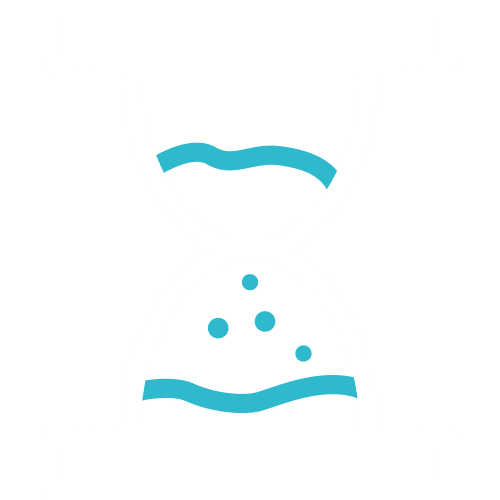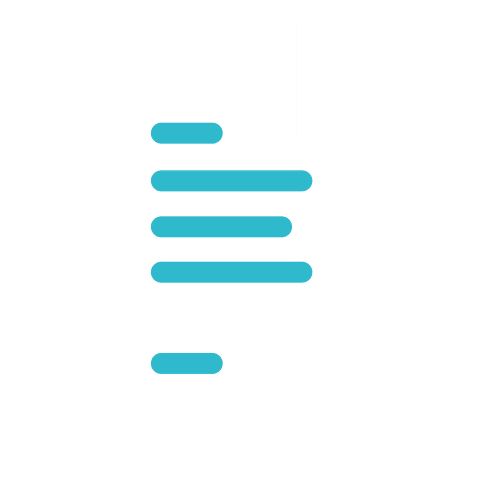# USEFUL FORMULAS & FUNCTIONS

## USEFUL FORMULAS & FUNCTIONS

### SummaryLocation

### MalaysiaDuration

2 DaysFormat

Public Class

Public Class

## Excel Training: Unlock Your Formula Superpowers

Are you ready to supercharge your Excel skills with top-notch Excel training? Dive into the world of ‘Useful Formulas & Functions’ and discover how to make Excel work for you like never before. Whether you’re an Excel novice or a seasoned pro, this course is designed to take your spreadsheet game to the next level.

### What You'll Learn

In this engaging course, you’ll master the art of Excel formulas and functions. From the basics of cell references to advanced functions like VLOOKUP and HLOOKUP, you’ll gain the skills to wrangle data effortlessly. With real-world examples and practical exercises, you’ll learn how to perform statistical calculations, manipulate text, and even work with dates and times like a pro. Our expert instructors will guide you through the world of array and database functions, giving you the tools to tackle complex data with ease.

### Why Choose Us

At Info Trek, we’ve been delivering top-notch corporate technology training for over two decades. We’re passionate about learning, innovation, and staying ahead in the fast-paced corporate world. Our Excel training isn’t just about formulas; it’s about empowering you to excel in your professional journey. Join us, and together, we’ll embrace new technology, discover efficient methods, and arm you with the knowledge to excel in the modern workplace.

Unleash the full potential of Excel with ‘Useful Formulas & Functions’—your gateway to becoming an Excel superhero. Sign up today and take the first step towards mastering the art of Excel.

### Course Details

Course Code: 50449; Instructor-led

#### Audience

This course is intended for users of Microsoft Office Excel who want to learn about useful formulas and functions.

#### Prerequisites

• Basic working knowledge of Microsoft Office Excel

#### Methodology

This program will be conducted with interactive lectures, PowerPoint presentations, discussions and practical exercises

#### Course Objectives

After completing this course, students will be able to:

• Apply Formula and Functions Basic
• Statistical and Logical Functions
• Lookup and Reference Formulas
• Text Formulas
• Date and Time Formulas
• Array and Database Functions
• Efficiency Tips

### Outlines

This module explains how to understand and apply Excel basic formulas and functions.

Lessons

• Formula basics
• Using cell references
• Copy formula without changing cell reference
• Transpose formula
• Using nested functions

After completing this module, students will be able to:

• Understand and apply formula basics
• Using cell references
• Copying formula without changing cell reference
• Transpose formula using paste special
• Using nested functions

This module explains how to use logical functions including CountIf, Sumif, If, IsError.

Lessons

• Perform calculation using CountIF
• Perform calculation using SumIF
• Perform calculation using AverageA
• Using IF function to prevent division by zero
• Using IsError function to avoid error display
• Creating multiple conditions using nested IF
• Using logical function OR, And

After completing this module, students will be able to:

• Perform calculation using CountIf, SumIf, AverageA
• Using If function to prevent division by zero
• Using IsError function to avoid error display
• Create multiple conditions using nested IF
• Using logical function OR, AND

This module explains how to apply and use lookup formulas including vlookup, hlookup, match and index.

Lessons

• Using Vlookup to find specific data
• Using Hlookup to find values in rows
• Using Match and Index to retrieve data
• After completing this module, students will be able to:
• Using Vlookup to find specific data
• Using Hlookup to find values in rows
• Using Match and Index to retrieve data

This module explains how to apply Text formula to help change casing of text, append text and numerical value in excel spreadsheet.

Lessons

• Changing case of text
• Append text and numerical value
• Convert imported text format into numbers
• Break imported date field into individual columns

After completing this module, students will be able to:

• Changing case of text using Upper, Lower or Proper formula
• Append text and numerical value
• Convert imported text format into numbers
• Break imported date field into individual columns

This module explains how to make use of calculate the difference of two given Date fields and to perform calculation with Time fields.

Lessons

• Perform addition to Date fields
• Calculate difference between two Dates
• Perform calculations with Time fields

After completing this module, students will be able to:

• Perform addition and calculate difference between two dates
• Perform calculations with Time fields

This module explains how to apply and use advance formula including Array, Frequency and Database functions.

Lessons

• Using Array Formulas
• Calculate the difference between Maximum and Minimum values
• Using Frequency function to Count responses
• Using Database functions DSum and DCount

After completing this module, students will be able to:

• Using Array Formulas
• Calculating the difference between Maximum and Minimum values in an Array
• Using Frequency function to Count responses in    tabulated data
• Using Database functions DSum and DCount

This module discusses some useful Excel Tips including application of Data Validations and Auditing Tools.

Lessons

• Shortening worksheets names
• Protecting cells containing formulas
• Using Data Validation
• Displaying Formula syntax
• Using Auditing Tools for errors checking
• Tracing precedent and dependent

After completing this module, students will be able to:

• Understand the advantages of shortening worksheet names
• Protecting cells from amendments by others
• Using Data validation to improve data entries
• Using Auditing Tools for checking errors
• Adding useful notes by commenting worksheet

Interested In

### USEFUL FORMULAS & FUNCTIONS

Starting From
RM1000
Intake Date
19-20 SEPT 2024, 7-8 NOV 2024, 14-15 MAR 2024, 20-21 MAY 2024, 9-10 JULY 2024, 11-12 JAN 2024
Class Type
Private, Public

## Book Now

Course Name: USEFUL FORMULAS & FUNCTIONS
Duration: 2 Days
Class Type *
Intake Date *
First Name *
Last Name *
Email *
Contact No. *
Pax *
Total Price: RM
0.00

Course Name *
Name *
Email *
Contact No. *
Enquiry For
Company Name *
Job Position *
Message *